﻿ 一类KdV型方程的新解析解 New Analytical Solutions of a Class of KdV-Type Equation

Vol.05 No.04(2016), Article ID:18926,7 pages
10.12677/AAM.2016.54069

New Analytical Solutions of a Class of KdV-Type Equation

Yifei Sun, Yu Pang

College of Sciences, North China University of Technology, Beijing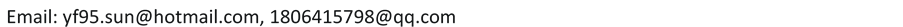Received: Oct. 18th, 2016; accepted: Nov. 7th, 2016; published: Nov. 11th, 2016ABSTRACT

We first use traveling wave reduction method to transform a class of KdV-type equation to ordinary differential equation, and then apply Exp-Function method as well as symbolic software Mathematica to obtain new accurate analytical solutions of the equation under study. Moreover, we draw the graphs of such solutions.

Keywords:Traveling Wave Reduction Method, Exp-Function Method, KDV-Type Equation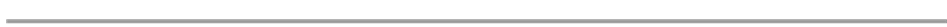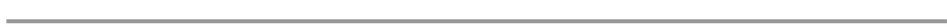1. 引言

1.1. 研究背景

1895年，荷兰的特韦格教授(Korteweg)和他的学生de Vries，在长近似波和小振幅的假设下，建立了单向浅水波的数学模型，从而得到了经典KDV Equation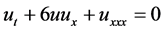。(1.1)

1.2. 本文研究的主要内容

2. 方法概述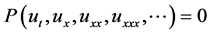(2.1)

1) 首先进行行波约化，假设方程(2.1)具有行波解：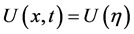，其中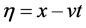(2.2)

2) 利用指数函数展开法求解非线性方程，假设(2.2)的方程具有如下形式：(2.3)

3) 将(2.3)代入到(2.2)中，然后平衡(2.2)中的非线性项和最高阶导数项的最高次数可以得到p和c的关系。同理，平衡非线性项和最高阶导数项的最低次数可以得到q和d的关系。

4) 对c，d，p和q赋特殊值，并将(2.3)代入(2.2)中可以得到关于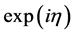的方程组。之后，令的系数为零，可得到一系列关于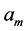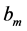的代数方程，求解这些代数方程并将结果代入(2.3)中，即可求得(2.1)的解。

3. 方程(1.1)求解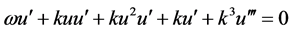(3.1)(3.2)(3.3)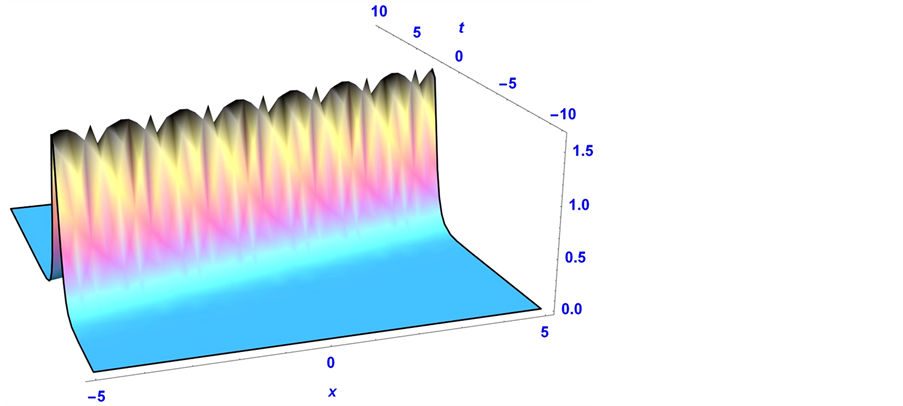Figure 1. Solution for Case 1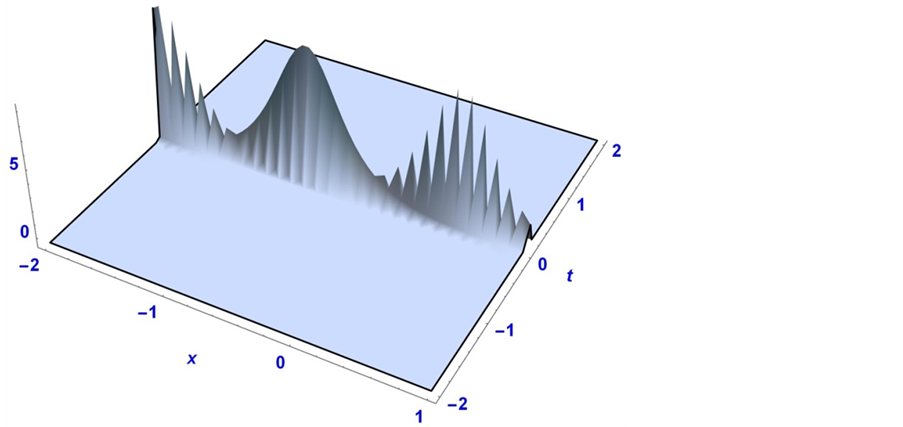Figure 2. Solution for Case 2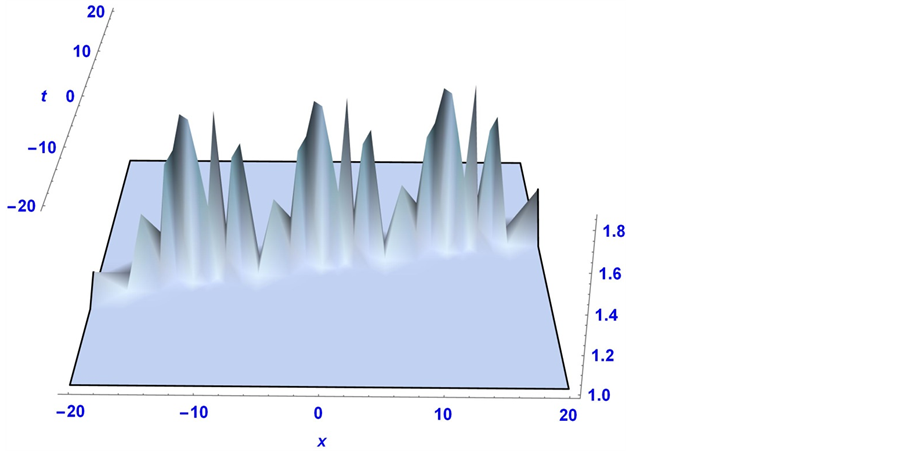Figure 3. Solution for Case 3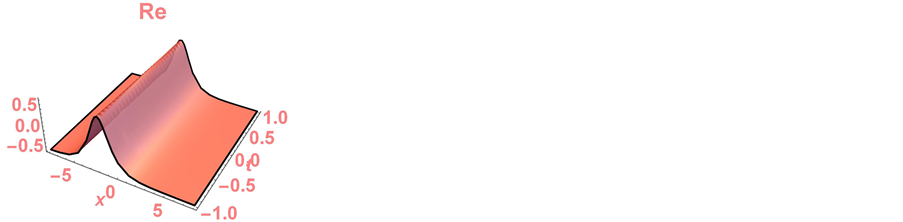Figure 4. The real part of solution for Case 4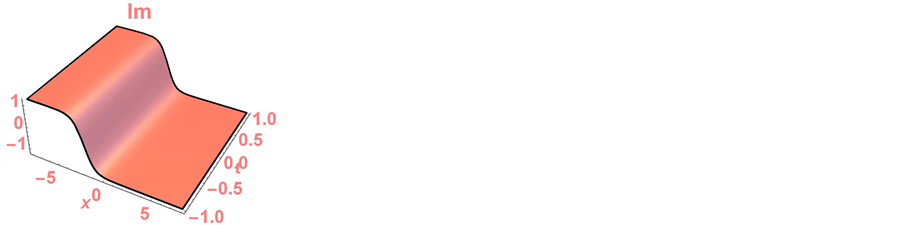Figure 5. The imaginary part of solution for Case 4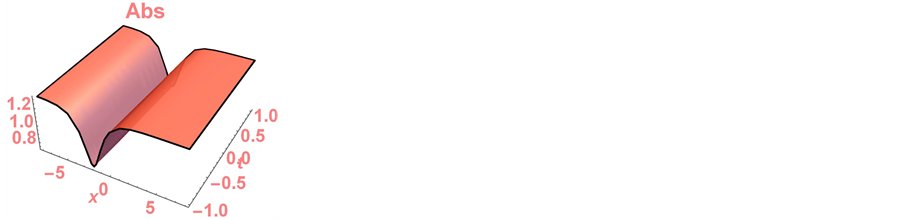Figure 6. The absolute value of solution for Case 4

4. 总结

New Analytical Solutions of a Class of KdV-Type Equation[J]. 应用数学进展, 2016, 05(04): 598-604. http://dx.doi.org/10.12677/AAM.2016.54069

1. 1. 王明亮, 李志斌, 周宇斌. 齐次平衡原则及其应用[J]. 兰州大学学报: 自然科学版, 1999(3): 8-16.

2. 2. 范恩贵, 张鸿庆. 非线性孤子方程的齐次平衡法[J]. 物理学报, 1998, 47(3): 353-362.

3. 3. 张金良, 王明亮, 王跃明. 推广的F-展开法及变系数KdV和mKdV的精确解[J]. 数学物理学报, 2006, 26(3): 353- 360.

4. 4. 刘式适, 刘式达, 傅遵涛, 等. Jacobi椭圆函数展开法及其在求解非线性波动方程中的应用[J]. 物理学报, 2001, 50(11): 2068-2073.

5. 5. Li, L.-X. and Wang, M.-L. (2009) The (G′/G)-Expansion Method and Travelling Wave Solutions for a Higher-Order Nonlinear Schrödinger Equation. Applied Mathematics & Computation, 208, 440-445. http://dx.doi.org/10.1016/j.amc.2008.12.005

6. 6. Zhang, S., Dong, L., Ba, J.M., et al. (2009) The (GG)-Expansion Method for Nonlinear Differential-Difference Equations. Physics Letters A, 373, 905-910. http://dx.doi.org/10.1016/j.physleta.2009.01.018

7. 7. Zhang, J., Wei, X. and Lu, Y. (2008) A Generalized -Expansion Method and Its Applications. Physics Letters A, 372, 3653-3658. http://dx.doi.org/10.1016/j.physleta.2008.02.027

8. 8. He, J.-H. and Wu, X.-H. (2006) Exp-Function Method for Nonlinear Wave Equations. Chaos, Solitons and Fractals, 30,700-708. http://dx.doi.org/10.1016/j.chaos.2006.03.020